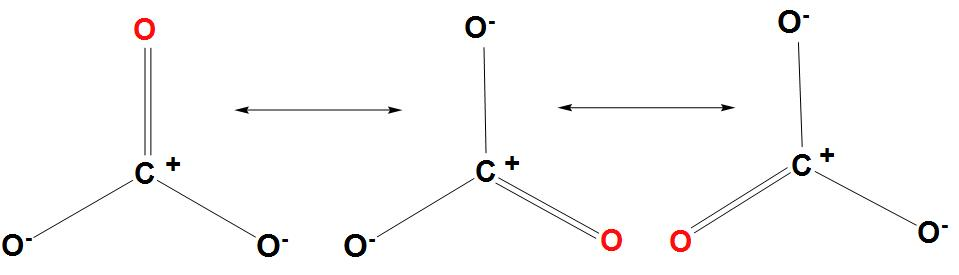QuestionAnswers

# How many resonance structures are there for $CO_{3}^{2-}$ ?Verified
91.8k+ views
Hint: As we know that resonance is the process of de-localization of the $\pi$- electrons that takes place in any compound which basically causes lowering of the energy of the compound and hence providing stability to it. And the energy which is released from the compound when it undergoes resonance is called resonance energy.

Complete Solution :
- It is found that in resonance a molecule can be expressed in different forms, and none of them can explain all the properties of the molecule. The main structure of the molecule is called a resonance hybrid.
- As we know that resonance is the extension of the fact that we can describe the bonding between the atoms in a chemical compound with the help of a Lewis structure.
- It is found that in some cases we can draw more than one Lewis structure, and the experimental properties are inconsistent with any one structure.
- In such a type of situation, some contributing structures are considered together as an average, and the molecule is represented by a resonance hybrid. It is found that in this several Lewis structures are used to describe the true structure.
- We can draw the resonance structures of $CO_{3}^{2-}$ as follows:- Hence we can conclude that three resonance structures are there for $CO_{3}^{2-}$.

Note: - As we know that resonance hybrid represents the main molecule as the average of the contributing structures. Resonance is basically a hypothetical phenomenon; it is actually impossible to say whether any molecule with a resonance structure is in one or another configuration.